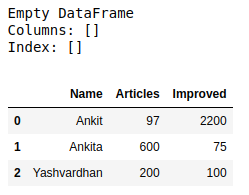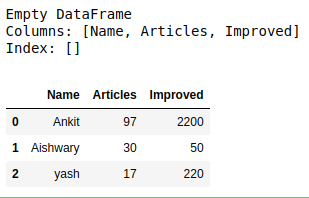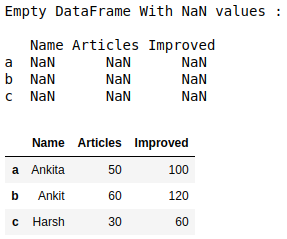# How to create an empty DataFrame and append rows & columns to it in Pandas?

## How to create an empty DataFrame and append rows & columns to it in Pandas?

Let’s discuss how to create an empty DataFrame and append rows & columns to it in Pandas. There are multiple ways in which we can do this task.

Method #1: Create a complete empty DataFrame without any column name or indices and then appending columns one by one to it.

 `# import pandas library as pd` `import` `pandas as pd` ` ` `# create an Empty DataFrame object` `df ``=` `pd.DataFrame()` ` ` `print``(df)` ` ` `# append columns to an empty DataFrame` `df[``'Name'``] ``=` `[``'Ankit'``, ``'Ankita'``, ``'Yashvardhan'``]` `df[``'Articles'``] ``=` `[``97``, ``600``, ``200``]` `df[``'Improved'``] ``=` `[``2200``, ``75``, ``100``]` ` ` `df`

Output:Method #2: Create an empty DataFrame with columns name only then appending rows one by one to it using `append()` method.

 `# import pandas library as pd` `import` `pandas as pd` ` ` `# create an Empty DataFrame` `# object With column names only` `df ``=` `pd.DataFrame(columns ``=` `[``'Name'``, ``'Articles'``, ``'Improved'``])` `print``(df)` ` ` `# append rows to an empty DataFrame` `df ``=` `df.append({``'Name'` `: ``'Ankit'``, ``'Articles'` `: ``97``, ``'Improved'` `: ``2200``}, ` `                ``ignore_index ``=` `True``)` `df ``=` `df.append({``'Name'` `: ``'Aishwary'``, ``'Articles'` `: ``30``, ``'Improved'` `: ``50``},` `                ``ignore_index ``=` `True``)` `df ``=` `df.append({``'Name'` `: ``'yash'``, ``'Articles'` `: ``17``, ``'Improved'` `: ``220``},` `               ``ignore_index ``=` `True``)` ` ` `df`

Output:Method #3: Create an empty DataFrame with a column name and indices and then appending rows one by one to it using `loc[]` method.

 `# import pandas library as pd` `import` `pandas as pd` ` ` `# create an Empty DataFrame object With` `# column names and indices ` `df ``=` `pd.DataFrame(columns ``=` `[``'Name'``, ``'Articles'``, ``'Improved'``], ` `                   ``index ``=` `[``'a'``, ``'b'``, ``'c'``])` ` ` `print``(``"Empty DataFrame With NaN values : \n\n"``, df)` ` ` `# adding rows to an empty ` `# dataframe at existing index` `df.loc[``'a'``] ``=` `[``'Ankita'``, ``50``, ``100``]` `df.loc[``'b'``] ``=` `[``'Ankit'``, ``60``, ``120``]` `df.loc[``'c'``] ``=` `[``'Harsh'``, ``30``, ``60``]` ` ` `df`

Output:Last Updated on October 24, 2021 by admin

## Python | Pandas dataframe.select_dtypes()Python | Pandas dataframe.select_dtypes()

Python | Pandas dataframe.select_dtypes() Python is a great language for doing data analysis, primarily because

## Python – Working with date and time using PandasPython – Working with date and time using Pandas

Python | Working with date and time using Pandas While working with data, encountering time

## Slicing, Indexing, Manipulating and Cleaning Pandas DataframeSlicing, Indexing, Manipulating and Cleaning Pandas Dataframe

Slicing, Indexing, Manipulating and Cleaning Pandas Dataframe With the help of Pandas, we can perform

## How to extract the value names and counts from value_counts() in Pandas ?How to extract the value names and counts from value_counts() in Pandas ?

How to extract the value names and counts from value_counts() in Pandas ? In this

## Pandas Series.cumsum() to find cumulative sum of a SeriesPandas Series.cumsum() to find cumulative sum of a Series

Python Pandas Series.cumsum() to find cumulative sum of a Series Pandas Series.cumsum() is used to find Cumulative

## Iterating over rows and columns in Pandas DataFrameIterating over rows and columns in Pandas DataFrame

Iterating over rows and columns in Pandas DataFrame Iteration is a general term for taking

## Python | Pandas Period.to_timestampPython | Pandas Period.to_timestamp

Python | Pandas Period.to_timestamp Python is a great language for doing data analysis, primarily because

## Python | Pandas dataframe.memory_usage()Python | Pandas dataframe.memory_usage()

Python | Pandas dataframe.memory_usage() Python is a great language for doing data analysis, primarily because